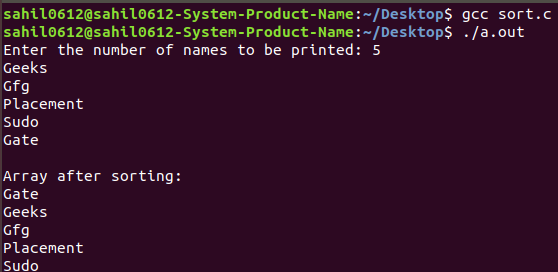# Sorting a dynamic 2-dimensional array of Strings

• Difficulty Level : Easy
• Last Updated : 10 Sep, 2018

Prerequisite: How to dynamically allocate a 2D array in C?

Double pointer: A pointer pointing to another pointer is known as a Double pointer. To represent the double pointer ‘ ** ‘ is used. Double pointer is also called as pointer to pointer.

Example:

```Input: Geeks, Gfg,  Placement, Sudo, Gate
Output: Gate, Geeks, Gfg, Placement, Sudo
```

The idea is to dynamically allocate memory and values to the strings in a form of a 2-D array. Then apply bubble sort using strcmp and strcpy function.

Below is the required implementation:

 `// C program to sort an array of strings``#include ``#include ``#include `` ` `// Function to sort the values``void` `sort(``char``** names, ``int` `n)``{``    ``int` `i, j;`` ` `    ``// Perform sort operation using bubble sort``    ``for` `(i = 0; i < n - 1; i++)``        ``for` `(j = 0; j < n - i - 1; j++)``            ``if` `(``strcmp``(names[j], names[j + 1]) > 0) {``                ``char``* temp;``                ``temp = (``char``*)``calloc``(30, ``sizeof``(``char``));``                ``strcpy``(temp, names[j]);``                ``strcpy``(names[j], names[j + 1]);``                ``strcpy``(names[j + 1], temp);``            ``}``}`` ` `// Driver code``int` `main()``{``    ``char``** names;``    ``int` `n, i;``    ``printf``(``"Enter the number of names to be printed: "``);``    ``scanf``(``"%d\n"``, &n);`` ` `    ``// allocating memory for 1st dimension``    ``names = (``char``**)``calloc``(n, ``sizeof``(``char``*));`` ` `    ``for` `(i = 0; i < n; i++)``    ``// allocating memory for 2nd dimension``    ``{``        ``names[i] = (``char``*)``calloc``(30, ``sizeof``(``char``));``        ``scanf``(``"%s"``, names[i]);``    ``}``    ``sort(names, n);`` ` `    ``printf``(``"\nArray after sorting:\n"``);``    ``for` `(i = 0; i < n; i++)``        ``printf``(``"%s\n"``, names[i]);`` ` `    ``return` `0;``}`

Output:My Personal Notes arrow_drop_up